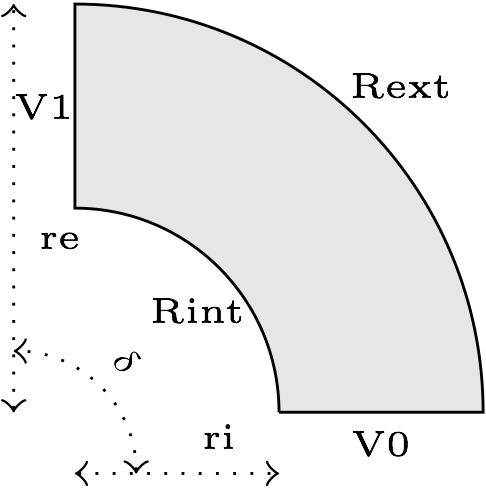# Quarter-Turn

In this example, we will estimate the current distribution in a stranded conductor. An electrical potential $V_0$ is applied to the entry/exit of the conductor.

## 1. Running the case

The command line to run this case in 2D is

``mpirun -np 4 feelpp_toolbox_electric --case "github:{path:toolboxes/electric/cases/cvg}" --case.config-file 2d.cfg``
Case option
`--case "github:{path:toolboxes/electric/cases/cvg}"`
Case config file option
`--case.config-file 2d.cfg`

The command line to run this case in 3D is

``mpirun -np 4 feelpp_toolbox_electric --case "github:{path:toolboxes/electric/cases/cvg}" --case.config-file 3d.cfg``
Case option
`--case "github:{path:toolboxes/electric/cases/cvg}"`
Case config file option
`--case.config-file 3d.cfg`

## 2. Geometry

The conductor consists in a rectangular cross section torus which is somehow "cut" to allow for applying electrical potential.+ In 2D, the geometry is as follow:In 3D, this is the same geometry, but extruded along the z axis.

## 3. Input parameters

Name Description Value Unit

$r_i$

1

$m$

$r_e$

2

$m$

$\delta$

angle

$\pi/2$

$rad$

$V_D$

electrical potential

9

$V$

### 3.1. Model & Toolbox

• This problem is fully described by an Electric model, namely a poisson equation for the electrical potential $V$.

• toolbox: electric

### 3.2. Materials

Name Description Marker Value Unit

$\sigma$

electric conductivity

omega

$4.8e7$

$S.m^{-1}$

### 3.3. Boundary conditions

The boundary conditions for the electrical probleme are introduced as simple Dirichlet boundary conditions for the electric potential on the entry/exit of the conductor. For the remaining faces, as no current is flowing througth these faces, we add Homogeneous Neumann conditions.

Marker Type Value

V0

Dirichlet

0

V1

Dirichlet

$V_D$

Rint, Rext, top*, bottom*

Neumann

0

*: only in 3D

## 4. Outputs

The main fields of concern are the electric potential $V$, the current density $\mathbf{j}$ and the electric field $\mathbf{E}$. // presented in the following figure.

## 5. Verification Benchmark

The analytical solution is given by:

\begin{align*} V&=\frac{V_D}{\delta}\theta=\frac{V_D}{\delta}\operatorname{atan2}(y,x)\\ \mathbf{E}&=\left( -\frac{V_D}{\delta}\frac{y}{x^2+y^2}, \frac{V_D}{\delta}\frac{x}{x^2+y^2}\right)\\ \end{align*}

We will check if the approximations converge at the appropriate rate:

• k+1 for the $L_2$ norm for $V$

• k for the $H_1$ norm for $V$

• k for the $L_2$ norm for $\mathbf{E}$ and $\mathbf{j}$

• k-1 for the $H_1$ norm for $\mathbf{E}$ and $\mathbf{j}$